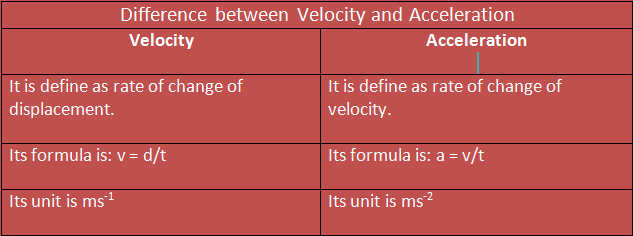Mechanics

# Difference between acceleration and velocityAcceleration Vs Velocity Acceleration and Velocity are two types of physical quantities. Acceleration is the rate of change of velocity,  while velocity is the displacement covered by unit time. SI unit of acceleration is m/s.s.
In this guide, you’ll learn about the difference between velocity and acceleration.
This post also contains lots of:

• Real-life examples
• Applications
• Much more

So, if you want to get benefits from this post, then you’ll love this post.
Let’s dive in…

## Acceleration Vs Velocity

 Difference between Acceleration and velocity Velocity Acceleration It is defined as the rate of change of displacement. It is defined as the rate of change of velocity. Its formula is: v = d/t Its formula is: a = v/t Its unit is ms-1 Its unit is ms-2

### What is the velocity?

The velocity is a  vector quantity and, as such, is represented by arrows indicating the direction and sense of movement that follows a body and whose length represents the numerical value. It depends on the displacement, that is, on the starting and ending points of the movement, and not on the speed, which depends directly on the trajectory.
Its unit of measurement in the International System (SI) is the meter per second (m / s),  this means that when for example we affirm that the speed (module) of a body is 5 meters per second (m / s), we are indicating that every second that same body moves 5 meters.

### What is acceleration?

The acceleration is the action and effect of accelerating (increasing speed). The term also allows us to name the vector magnitude that expresses said an increase in speed in a unit of time (meter per second every second, according to its unit in the International System).
Acceleration can be negative; in these cases, the magnitude would express a decrease in speed as a function of time.
It is important to distinguish between velocity (which reflects how a body’s position changes with respect to time) and acceleration (which shows how velocity has varied). Acceleration mentions how velocity changes, not what velocity is like: a body moving at high velocity can have very little acceleration.
There are different types of acceleration. The tangential acceleration is one that relates to the change in speed over time. On the other hand, normal or centripetal acceleration links changes in direction over time.
On the other hand, the average acceleration allows calculating the average change of speed in a certain time interval.
All of these terms are very important, as is the well-known centrifugal acceleration, which is that which is irremediably determined by the centrifugal force. That is, it is the acceleration that moving bodies experience within a rotation system and that means that they will “flee” from the center.
And all this without forgetting what is known as Coriolis acceleration or Coriolis effect. The French scientist who gives it its name, Gaspard-Gustave Coriolis, was the one who in 1836 described that which is nothing more than the relative acceleration of a body in motion within what is a rotation reference system.
Acceleration can have different directions. When the body is slowing down, its acceleration is directed in the opposite direction of its movement. On the other hand, when a body is increasing its speed (that is, it is accelerating), acceleration maintains the same direction as speed.
In addition to all the above, we cannot ignore the fact that there is also what is known as the acceleration of the fixed stars. An expression is used in the field of Astronomy to refer to the daily interval in which, by the same meridian, the passage of a star is ahead of that carried out by the Sun. Specifically, that has been calculated to correspond to three minutes and fifty-six seconds.
Related Topics: